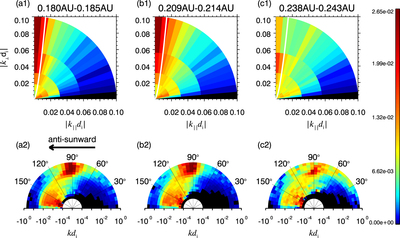Image Details

Choose export citation format:Wave Composition, Propagation, and Polarization of Magnetohydrodynamic Turbulence within 0.3 au as Observed by Parker Solar Probe

• Authors: Xingyu Zhu, Jiansen He, Daniel Verscharen, Die Duan, and Stuart D. Bale

2020 The Astrophysical Journal Letters 901 L3.

• Provider: AAS Journals

Caption: Figure 2.

Panels (a1), (b1), and (c1): PDFs of the wavevector in ﹩| {k}_{\parallel }{d}_{{\rm{i}}}| -| {k}_{\perp }{d}_{{\rm{i}}}| ﹩ space in the range of 0.180 au–0.185 au, 0.209 au–0.214 au, and 0.238 au–0.243 au, respectively. The white solid lines represent the relation between k and k as predicted from the phenomenology of critical balance, ﹩{k}_{\parallel }\sim {k}_{\perp }^{\tfrac{2}{3}}{k}_{0}^{\tfrac{1}{3}}﹩, where k0 is the wavenumber of the outer scale. Panels (a2), (b2), and (c2): PDFs of the propagation angle (﹩{\theta }_{{\rm{k}},{{\rm{B}}}_{0}}﹩) at differing scales (kdi), in the corresponding distance ranges.